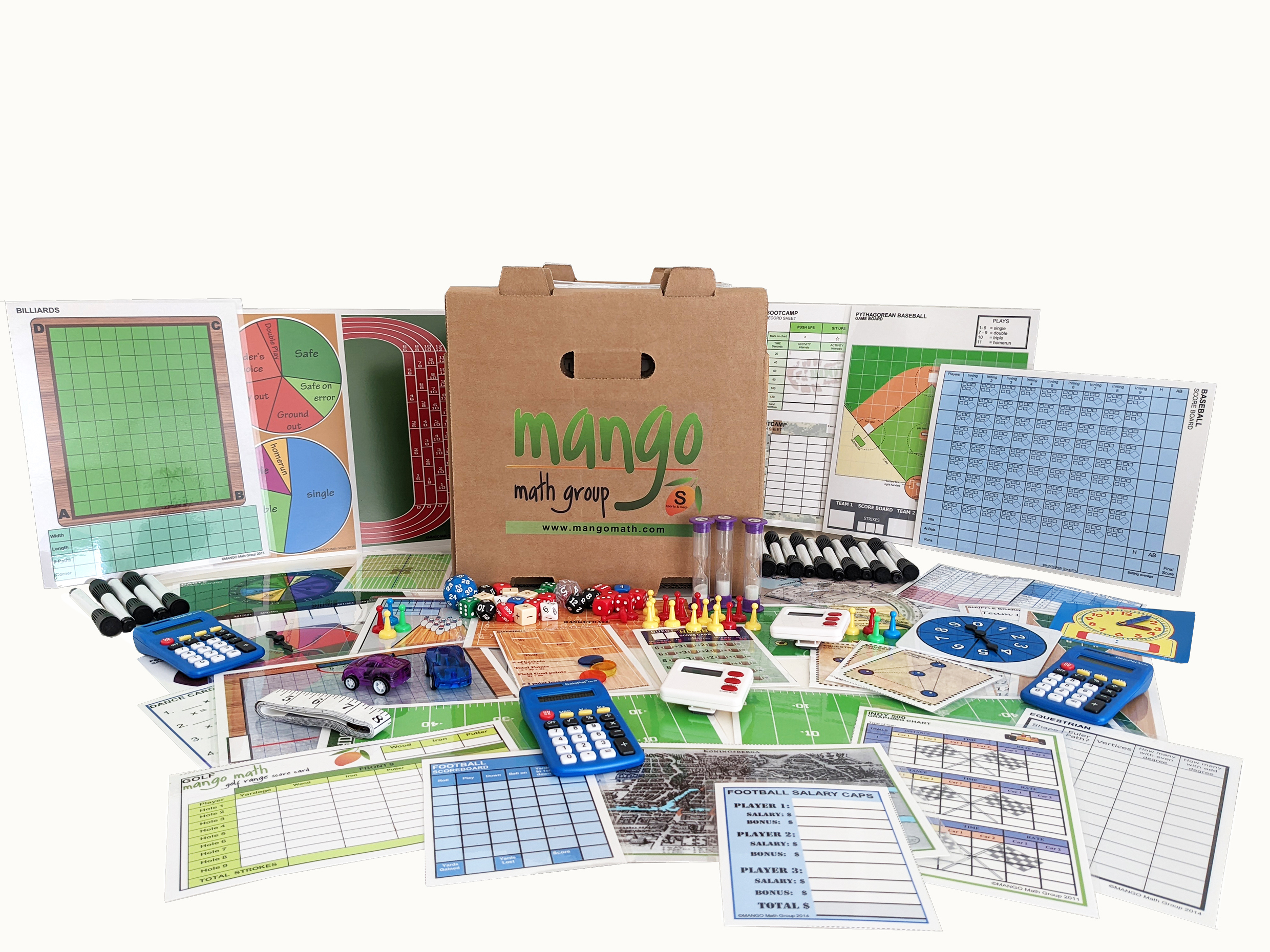#### Order Notice

As the economy opens back up, many of our suppliers are experiencing shortages of parts and raw materials, resulting in delays in shipments. As a result, we anticipate that it may take up to 2-4 weeks from the date we receive a purchase order for us to fulfill and ship any order for our products. We apologize for any inconvenience.

NEW!!

# Deluxe Math and Sports Kit

What better way to explore math than through sports! MANGO Math Sports and Math kit is designed specifically for grades 5-6-7-8. The kit contains 20 separate skill building math lessons.

\$ 479.00 USD##### Product Details

What better way to explore math than through sports! MANGO Math Sports and Math kit is designed specifically for grades 5-6-7-8. The kit contains 20 separate skill building math lessons.

Each individual lesson offers visual and kinetic representation of math concepts that develop strong math knowledge, like:

• ratio
• fractions
• percent
• integers expressions
• factors
• exponents

There are 17 separate sports from traditional Football and Basketball to Equestrian and Dance and much more. Every lesson is based on a game or activity that fosters curiosity, discussion and growth through cooperation, collaboration and creative thinking.

MANGO Math's Strong Evidence Based Kits come with individually packaged, Grab-n-Go lessons that includes instructions, reusable materials pre-packaged in a resealable pouch contained in an easy to carry crate that can be used year after year, providing long term savings.

A lesson can be used by up to 4 students, set up as math centers with each grade level math kit meeting the needs of 30+ students at a time.

Each MANGO Math grade level math kit is research-based and adheres to Common Core, TEKS and NCTM math standards.

Great to use in regular classrooms, resource rooms, after school programs, STEM programs, libraries, math centers, summer camps, learning cooperatives, math clubs, community centers, and at home.

#### All the supplies below are included in this pack:

• Patten blocks
• Connecting cubes
• Plastic fruit counters
• MANGO Math playing cards
• Clothes pins
• 10s dice
• 100s dice
• 10-sided dice
• 30-sided dice
• Game pawns
• Play money
• Positive / negative dice
• Numbered dice
• Stacking counters
• Plastic resealable pouches
• Game boards
• Laminated writing boards
• Dry erase markers
• Easy to follow directions
• Curriculum guide

## Lessons and Standards

Horsing Around – Equestrian - Mathematical Thinking: Using a course that a horse might follow students will apply mathematical practices of reasoning, argument, and perseverance to solve problems.

Billiards – Ratio: Using a pool/billiard table to understand the concept and use ratio language to describe a ratio relationship between two quantities.

Dance Equation – Geometry: Write expressions that record operations with numbers and with letters standing for numbers. Describe the effects of translations, rotations, and reflections on figures.

Doggone It – Iditarod Dog Sled Race – Measurement: Using the Iditarod race as a means to solve real world math problems dealing with elapsed time.

Hoopfest – Basketball – Expressions: Using basketball scores to solve real-world and mathematical problems leading to two linear equations in two variables.

Indy 500 – Car Race – Expressions & Equations: Use variables to represent two quantities in a real-world problem that changes in relationship to one another. Compare two proportional relationships.

Salary Caps – Football – Proportions: Solve real world problems using proportional relationships to solve multistep ratio and percent problems.

Score Board – Football – Expressions: Understanding that rewriting an expression in different forms in a problem context can shed light on the problem and how the quantities in it are related.

Throwing Strikes – Bowling – Operations & Algebraic Thinking: Using bowling to think flexibly about numbers and operations. Record multiple operations using proper notation. Write and interpret numerical expression.

Batter Up – Baseball – Ratio & Proportions: Use proportional relationships to solve multi-step ratio and percent problems

Bulls-eye – Darts – Expressions & Equations: Using a dart game to practice writing, reading and evaluating expressions in which letters stand for numbers.

Gaining Yardage – Football – Number Sense: Using a football field understand p + q as the number located a distance q from p, in the positive or negative direction depending on whether q is positive or negative.

Keeping Track – Track & Field – Number Sense: Add fractions with unlike denominators by replacing given fractions with equivalent fractions in such a way as to produce an equivalent sum with like denominators.

Par for the Course – Golf – Expression & Equations: Using a golf course students will understand that positive and negative numbers are used together to describe quantities having opposite direction.

Shuffling – Shuffleboard – Ratio & Number Sense: Solve real world problems involving multiplication of fractions by using visual fraction models. Understand the concept of a ratio and use ratio language to describe a ratio relationship between two quantities.

Full of Hot Air – Hockey – Geometry: Use facts about angles to solve simple equations for an unknown angle in a figure.

Marching On – Marching Band – Geometry: Describe the effect of dilations, translations, rotations, and reflections on two-dimensional figures using coordinates.

Pythagorean Baseball – Geometry: Using a baseball field, student will apply the Pythagorean Theorem to determine unknown side lengths in right triangles in re-world problems.

Soccer Kicks – Geometry: Using a soccer game to understand how to reason abstractly and construct viable arguments while maintaining precision. Using geometrical terms.

Scatter Plot Boot Camp – Statistics: Using a physical workout, students will construct and interpret scatter plots for bivariate measurement data to investigate patterns of association between two quantities.

Width
13
in
Height
13
in
Length
38
in
Weight
40
lb